### Artificial Neural Networks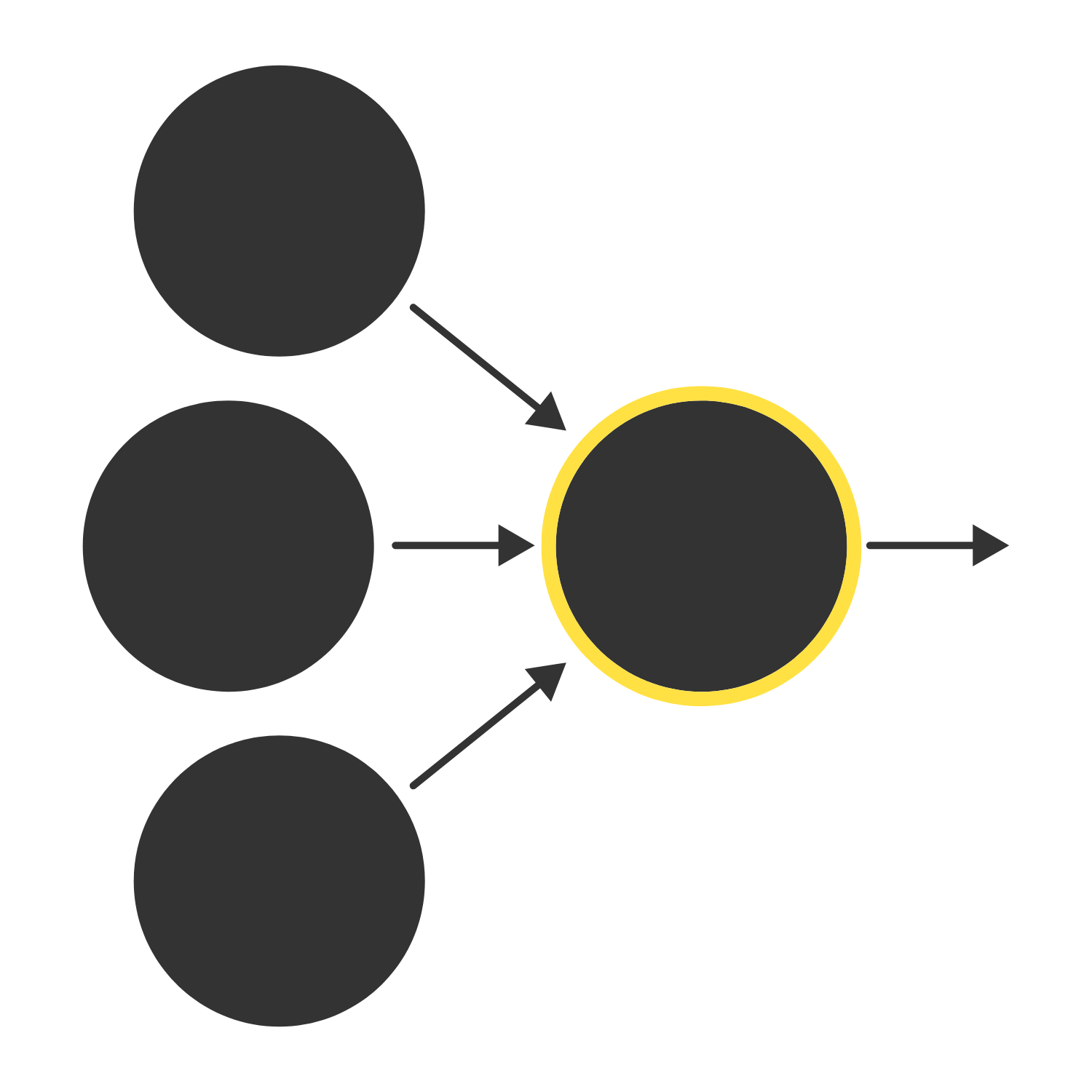A neuron has many inputs but only one output, so it must "integrate" its inputs into one output (a single number). Recall that the inputs to a neuron are generally outputs from other neurons. What is the most natural way to represent the set of these inputs to a single neuron in an ANN?

# Computational Models of The Neuron

In our computational model of a neuron, the inputs defined by the vector $\vec{x}$ are “integrated” by taking the bias $b$ plus the dot product of the inputs $\vec{x}$ and weights $\vec{w}:$ $\vec{w} \cdot \vec{x} + b.$ The dot product represents a "weighted sum" because it multiplies each input by a weight.

A biological interpretation is that the inputs defining $\vec{x}$ are the outputs of other neurons, the weights defining $\vec{w}$ are the strengths of the connections to those neurons, and the bias $b$ impacts the threshold the computing neuron must surpass in order to fire.

# Computational Models of The Neuron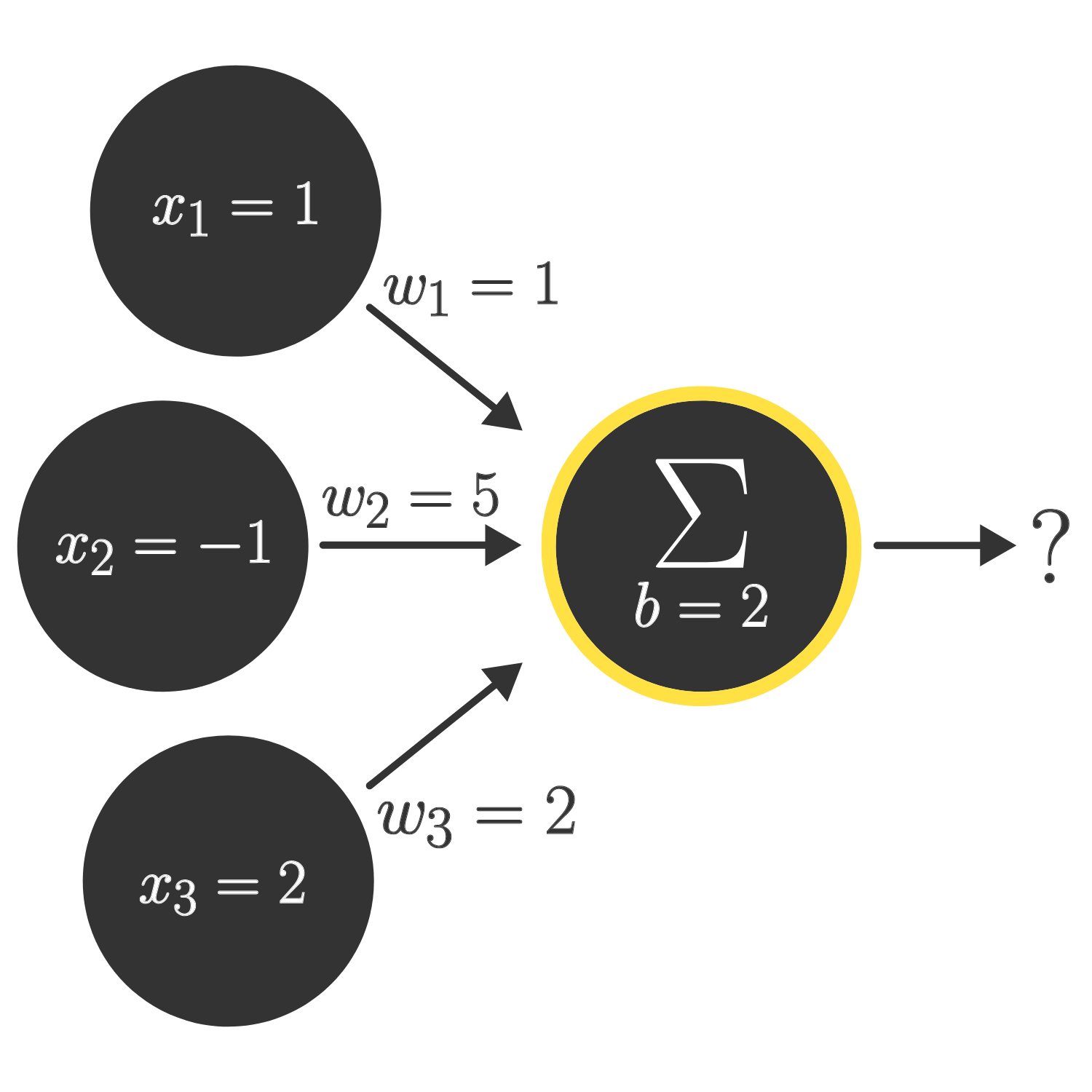Given the inputs, weights, and bias shown above, what is the integration of these inputs given by the weighted sum $\vec{w} \cdot \vec{x} + b?$

Note: If you are unfamiliar with dot products, our wiki on the dot product in Cartesian coordinates might be helpful.

# Computational Models of The Neuron

An activation function, $H(v),$ is used to transform the integration (weighted sum) into a single output which determines whether or not the neuron would fire. For example, we might have $H(v)$ as the Heaviside step function, that is, $H(v) = \begin{cases} 1 & \mbox{if } v \ge 0 \\ 0 & \mbox{if } v \lt 0. \\ \end{cases}$ Considering $H(\vec{w} \cdot \vec{x} + b),$ how does increasing the bias $b$ affect the likelihood of the neuron firing (all else equal), assuming that a $1$ corresponds to firing?

# Computational Models of The Neuron

When $H(v)$ is the Heaviside step function, the neuron modeled by $H(\vec{w} \cdot \vec{x} + b)$ fires when $\vec{w} \cdot \vec{x} + b\ge 0.$

The hypersurface $\vec{w} \cdot \vec{x} + b = 0$ is called the decision boundary, since it divides the input vector space into two parts based on whether the input would cause the neuron to fire. This model is known as a linear classifier because this boundary is based on a linear combination of the inputs.

# Computational Models of The Neuron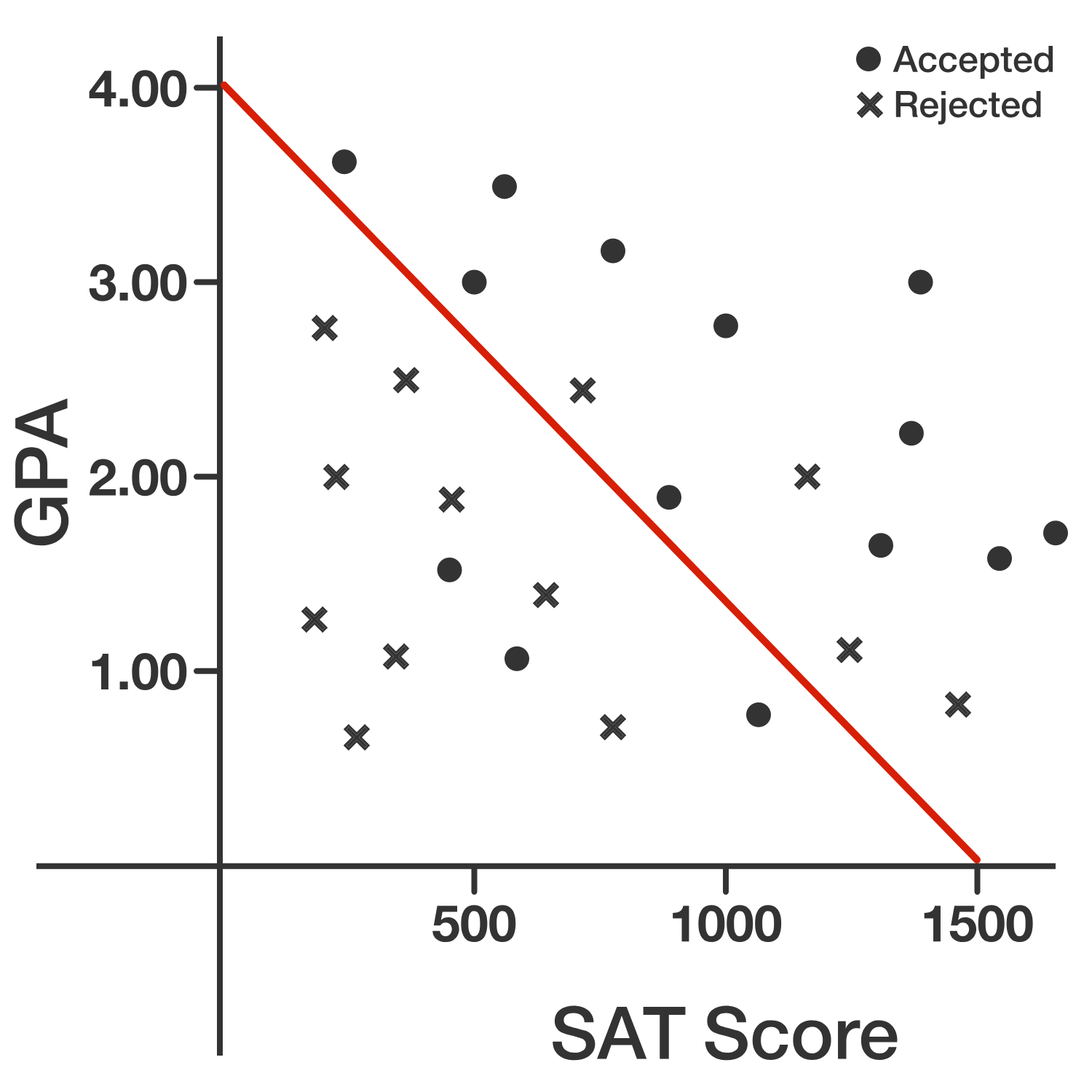The model above shows a decision boundary for predicting college admission based on the input $\vec{x} = \begin{pmatrix}\text{SAT score} \\ \text{GPA} \end{pmatrix}$ and the activation function $H(\vec{w} \cdot \vec{x} + b)$, where $H(v)$ is the Heaviside step function. Which of the following is a possible value for the weight vector, $\vec{w}?$

# Computational Models of The Neuron

So far, we’ve considered an activation function $H(v)$ with binary outputs, as inspired by a physical neuron. However, in ANNs, we don’t need to restrict ourselves to a binary function. Functions like the ones below avoid counterintuitive jumps and can model continuous values (e.g. a probability):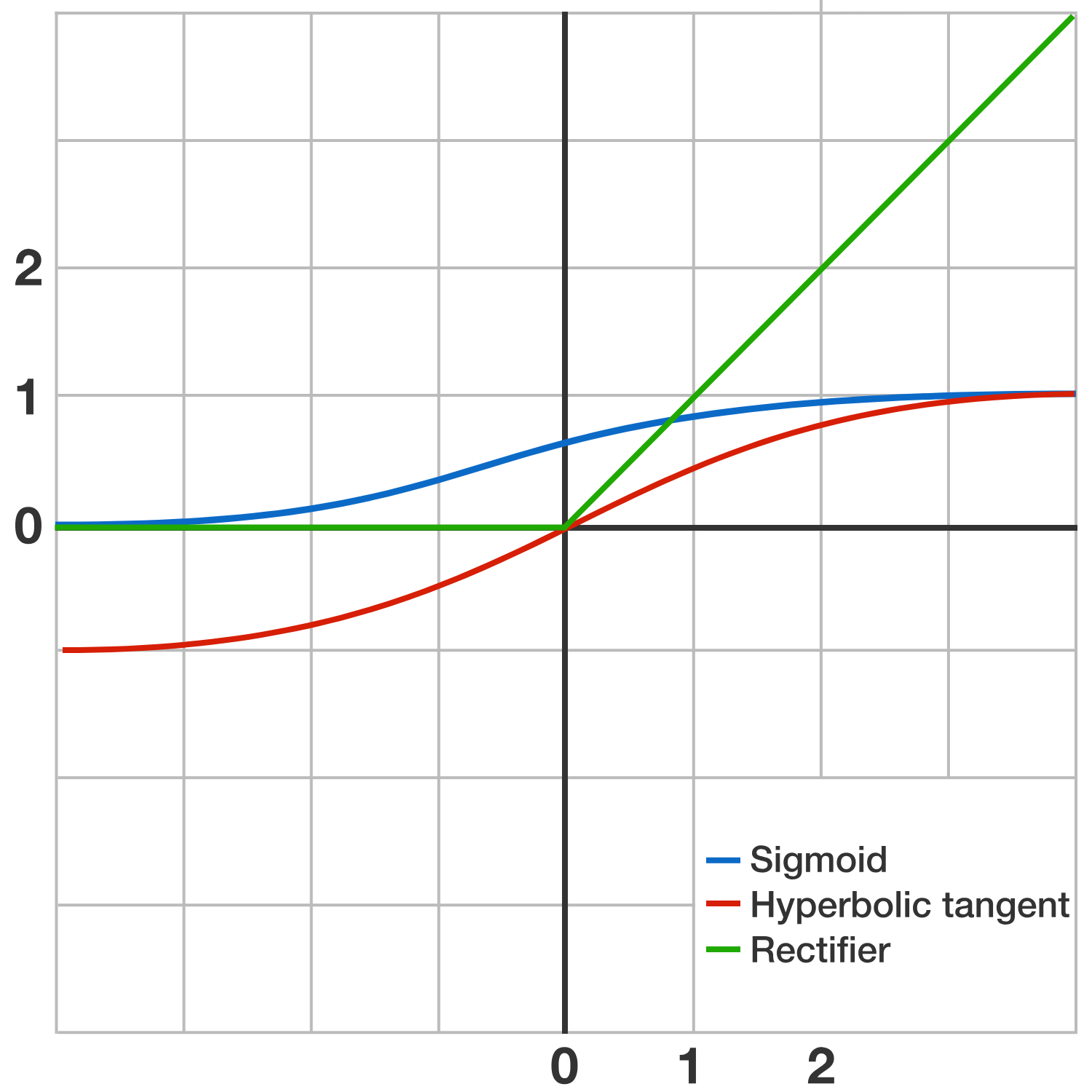The power of ANNs is illustrated by the universal approximation theorem, which states that ANNs using activation functions like these can model any continuous function, given some general requirements about the size and layout of the ANN.

We can't prove the universal approximation theorem here, but its implications are still important. No matter how complicated a situation is, a sufficiently large ANN with the appropriate parameters can model it.

# Computational Models of The Neuron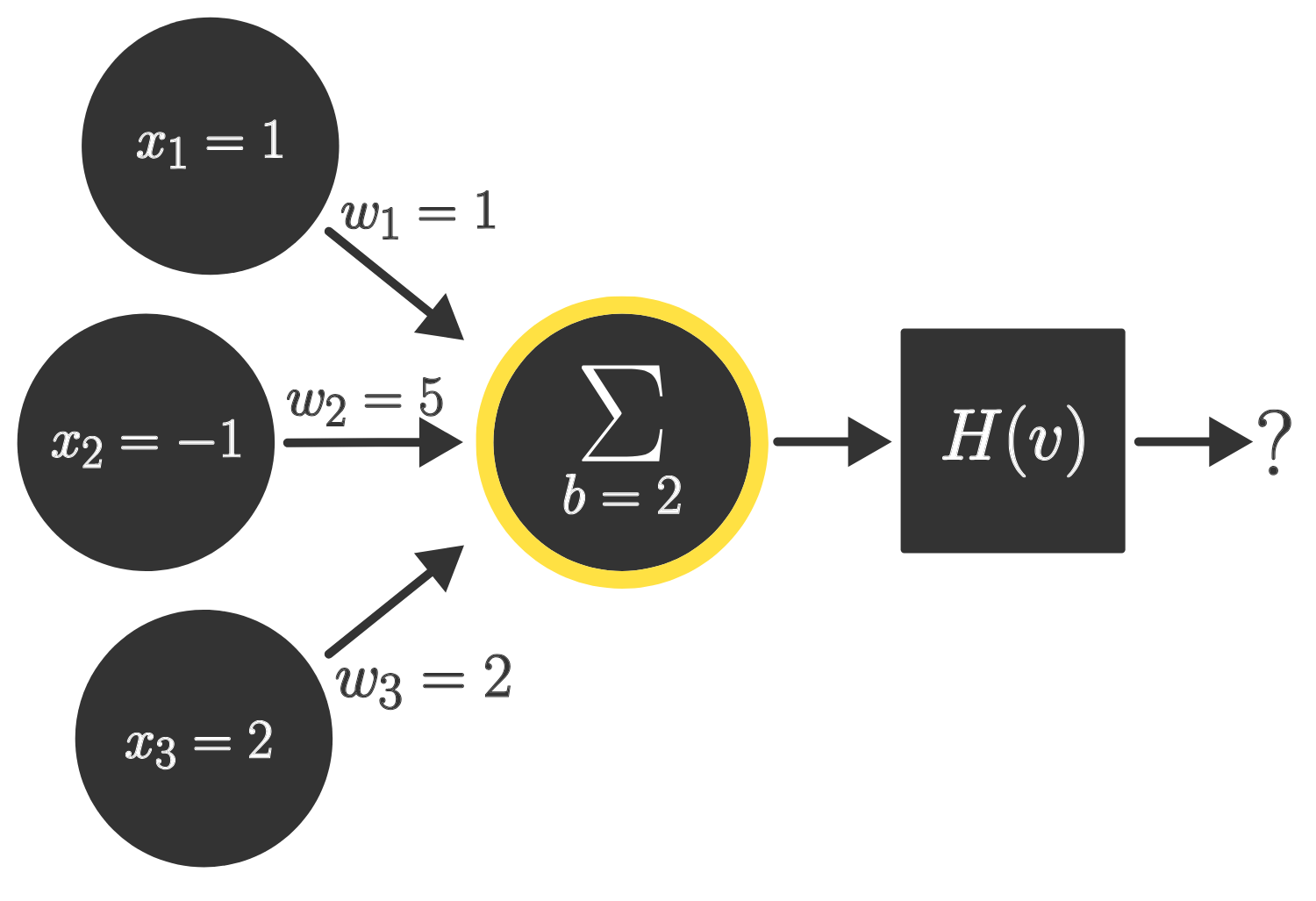Consider the activation function $H(v) = \dfrac{1}{1+e^{-v}}$, where $e$ stands in for Euler's Number, $2.71828\ldots$

$H(v)$ is known as the sigmoid function. In our image above, we multiply our inputs by their corresponding weights and add a bias of $2$ to get $v$. Then the value in $v$ is fed into the activation function to get the output of the neuron.

Given the inputs, weights, and bias shown in the image above (which are the same as in an earlier question), what is the approximate output (to the nearest thousandth) from this neuron after the integrated value of the inputs is evaluated by the activation function?

# Computational Models of The Neuron

We’ve now built up a basic computational model of neurons. While one neuron might not seem powerful, connecting many together in a clever manner can yield a highly effective learning model. This turns out to be true for ANNs, as evidenced by the universal approximation theorem.

The remainder of this course focuses on the methods used to construct and train ANNs, highlighting the intuition behind the models and their applications. Let’s dive in!

# Computational Models of The Neuron

×